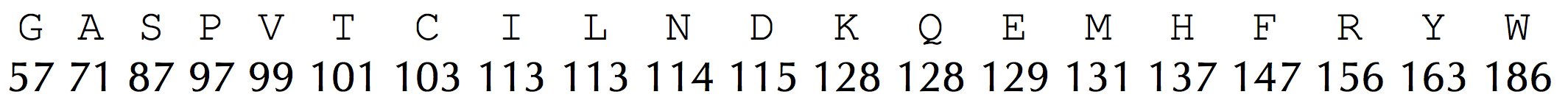# Generate the Theoretical Spectrum of a Linear Peptide solved by 400

Sept. 7, 2015, 4:11 p.m. by Rosalind TeamFigure 1. The arrays AminoAcid (top row) and AminoAcidMass (bottom row) corresponding to the integer mass table.

Given an amino acid string Peptide, we will begin by assuming that it represents a linear peptide. Our approach to generating its theoretical spectrum is based on the assumption that the mass of any subpeptide is equal to the difference between the masses of two prefixes of Peptide. We can compute an array PrefixMass storing the masses of each prefix of Peptide in increasing order, e.g., for Peptide = NQEL, PrefixMass = (0, 114, 242, 371, 484). Then, the mass of the subpeptide of Peptide beginning at position i + 1 and ending at position j can be computed as PrefixMass(j) − PrefixMass(i). For example, when Peptide = NQEL,

Mass(QE) = PrefixMass(3) − PrefixMass(1) = 371 − 114 = 257.

The pseudocode shown on the next step implements this idea. It also represents the alphabet of 20 amino acids and their integer masses as a pair of 20-element arrays AminoAcid and AminoAcidMass, corresponding to the top and bottom rows of the following integer mass table, respectively.

Figure 1

LinearSpectrum(Peptide, AminoAcid, AminoAcidMass)    PrefixMass(0) ← 0    for i ← 1 to |Peptide|        for j ← 1 to 20            if AminoAcid(j) =  i-th amino acid in Peptide                PrefixMass(i) ← PrefixMass(i − 1) + AminoAcidMass(j)    LinearSpectrum ← a list consisting of the single integer 0
for i ← 0 to |Peptide| − 1        for j ← i + 1 to |Peptide|            add PrefixMass(j) − PrefixMass(i) to LinearSpectrum    return sorted list LinearSpectrum

# Linear Spectrum Problem

Generate the ideal linear spectrum of a peptide.

Given: An amino acid string Peptide.

Return: The linear spectrum of Peptide.

## Sample Dataset

NQEL


## Sample Output

0 113 114 128 129 242 242 257 370 371 484